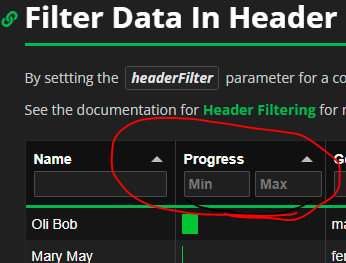# Dynamic column name in `add_filter` method of tabulator

Hi,
I tried to modify the tabulator filtering example by replacing the column string argument with the widget (to be able to filter value ranges of a dynamically selected column):

``````import pandas as pd
import panel as pn
pn.extension('tabulator')
mixed_df = pd._testing.makeMixedDataFrame()
filter_table = pn.widgets.Tabulator(mixed_df)
slider = pn.widgets.RangeSlider(start=0, end=5, name='A Filter')
select = pn.widgets.Select(value = 'A', options=mixed_df.select_dtypes(include=["float64","int64"]).columns.to_list(), name='C Filter')

pn.Row(
pn.Column(slider, select),
filter_table
)
``````

Unfortunately it looks like this is not implemented. Is there any way to achieve this functionality? I tried to do it with `pn.bind` or `@pn.depends` but wasn´t successful. I´m not very experienced and might have a knot in my brain.
I finally could do it by modifying `add_filter` method directly in tables.py and modifying the `col_name` to `column = df[col_name.owner.value]` in `_filter_dataframe` (it´s not very clean programming and I´m not sure if I can do this in a general way for everyone but it solves my problem for the moment and might help for future implementation, so, here is the code:)

``````    def add_filter(self, filter, column=None):
#######MODIFIED PM###################
if isinstance(filter, (tuple, list, set)) or np.isscalar(filter):
deps = []
elif isinstance(filter, (FunctionType, MethodType)):
deps = list(filter._dinfo['kw'].values()) if hasattr(filter, '_dinfo') else []
else:
filter = param_value_if_widget(filter)
column = param_value_if_widget(column)
if not isinstance(filter, param.Parameter):
raise ValueError(f'{type(self).__name__} filter must be '
'a constant value, parameter, widget '
'or function.')
elif column is None:
raise ValueError('When filtering with a parameter or '
'widget, a column to filter on must be '
'declared.')
deps = [filter]
deps2 = [column]

for dep in deps:
dep.owner.param.watch(self._update_cds, dep.name)

for dep2 in deps2:
dep2.owner.param.watch(self._update_cds, dep2.name)
self._filters.append((column, filter))
self._update_cds()
#######MODIFIED PM###################
``````

On the other hand I think the client side filtering is very attractive, as the input fields are directly above the column. This works great for strings or “>” “<” filtering but not for ranges.
Here it would be great to find a way to implement the min/max input field from:Because you can pass a function to `add_filter`, and in particular a bound function (bound with `pn.bind`), it’s really flexible and you can achieve the filtering on a given column you want:

``````import pandas as pd
import panel as pn

pn.extension('tabulator')

mixed_df = pd._testing.makeMixedDataFrame()
table = pn.widgets.Tabulator(mixed_df)

slider = pn.widgets.RangeSlider(start=0, end=5, name='A Filter')
select = pn.widgets.Select(
value='A',
options=mixed_df.select_dtypes(include=["float64","int64"]).columns.to_list(),
name='C Filter'
)

def filter_table(df, column, rng):
return df[(rng <= df[column]) & (df[column] <= rng)]

pn.Row(
pn.Column(slider, select),
table
).servable()
``````
1 Like

As for the min-max header filter of the Tabulator JS library, if you have a look at the source code of the example (Tabulator) you’ll see that it’s a very customized one, created by hand, so it’s not just a parameter Panel would have to set. I agree it looks awesome though, it’d probably be first a feature request to Tabulator itself before being able to have it in Panel.

1 Like

Thank you very much!
The thing that confused me was the return value of the pn.bind being a dataframe and not (filter,column). But obviously it works like the function based filtering example. Sorry I couldn’t put 1and1 together.

As for min/max in Tabulator I posted a feature request,let’s see .

1 Like